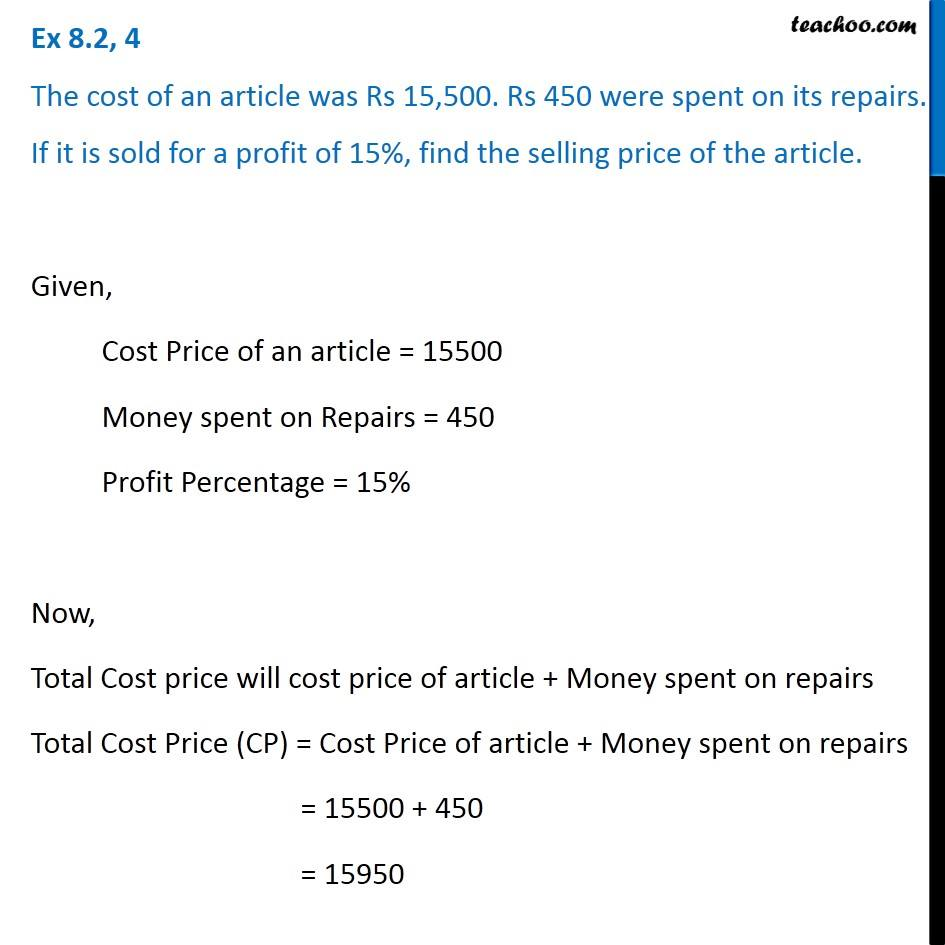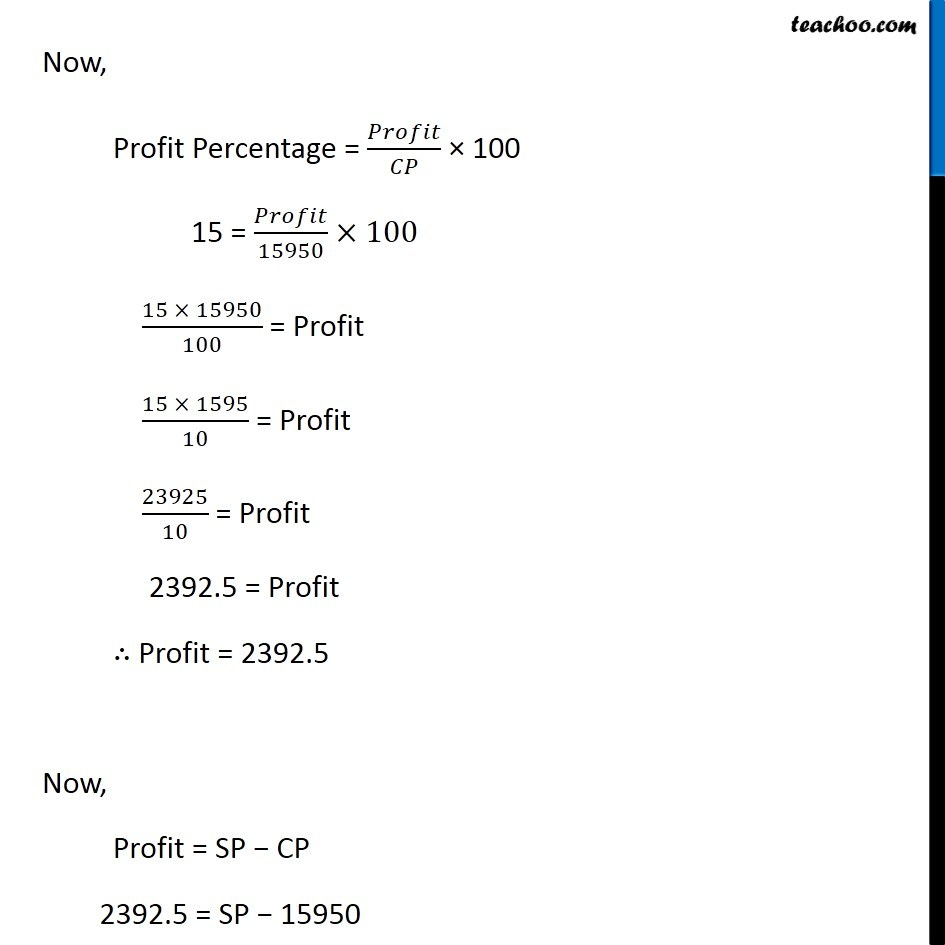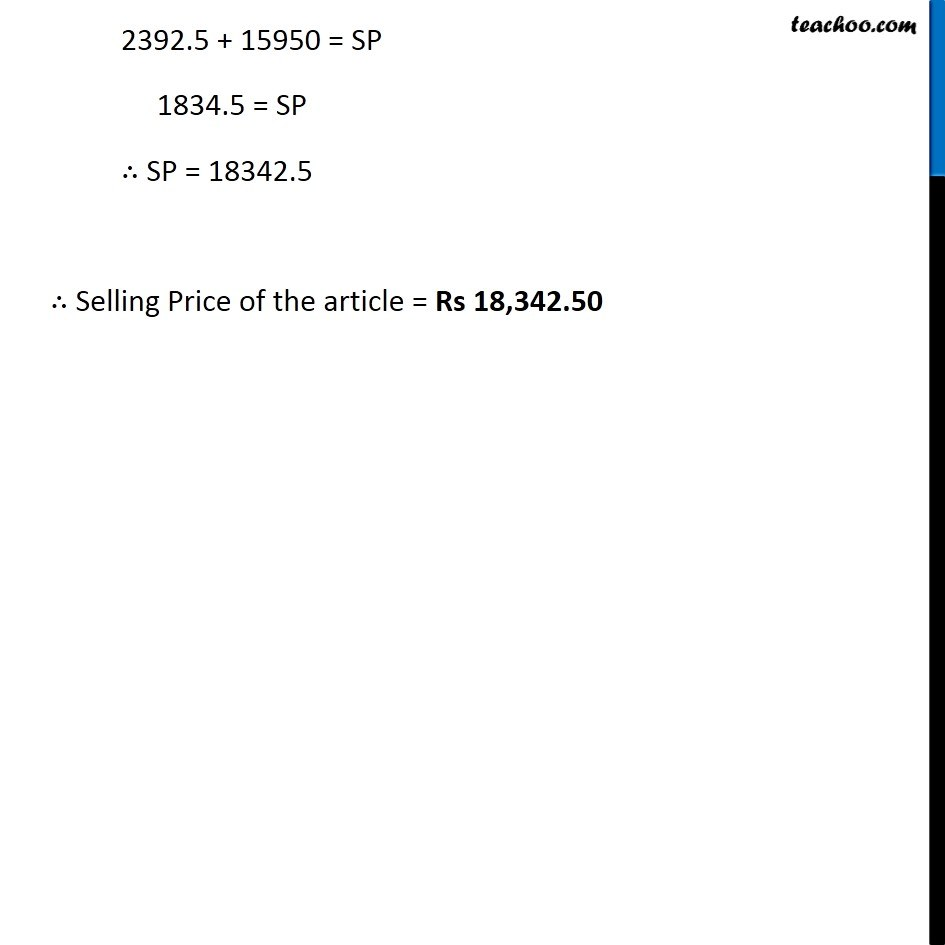Finding cost price, selling price, profit % , loss%

Chapter 8 Class 8 Comparing Quantities
Concept wiseIntroducing your new favourite teacher - Teachoo Black, at only ₹83 per month

### Transcript

Ex 8.2, 4 The cost of an article was Rs 15,500. Rs 450 were spent on its repairs. If it is sold for a profit of 15%, find the selling price of the article. Given, Cost Price of an article = 15500 Money spent on Repairs = 450 Profit Percentage = 15% Now, Total Cost price will cost price of article + Money spent on repairs Total Cost Price (CP) = Cost Price of article + Money spent on repairs = 15500 + 450 = 15950 Now, Profit Percentage = 𝑃𝑟𝑜𝑓𝑖𝑡/𝐶𝑃 × 100 15 = 𝑃𝑟𝑜𝑓𝑖𝑡/15950×100 (15 × 15950)/100 = Profit (15 × 1595)/10 = Profit 23925/10 = Profit 2392.5 = Profit ∴ Profit = 2392.5 Now, Profit = SP − CP 2392.5 = SP − 15950 2392.5 + 15950 = SP 1834.5 = SP ∴ SP = 18342.5 ∴ Selling Price of the article = Rs 18,342.50